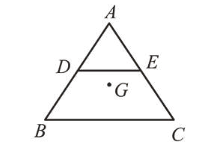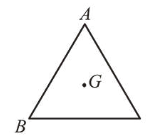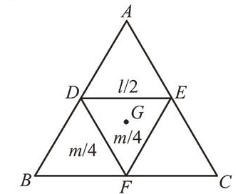# A B C is a plane lamina of the shape of an equilateral triangle.Question:

$A B C$ is a plane lamina of the shape of an equilateral triangle. $D, E$ are mid points of $A B, A C$ and $G$ is the centroid of the lamina. Moment of inertia of the lamina about an axis passing through $G$ and perpendicular to the plane $A B C$ is $I_{0}$. If part $A D E$ is removed, the moment of inertia of the

remaining part about the same axis is $\frac{N I_{0}}{16}$ where $N$ is an integer. Value of $N$ is______Solution:

(11)

Let mass of triangular lamina $=m$, and length of side $=l$, then moment of inertia of lamina about an axis passing through centroid $G$ perpendicular to the plane.

$I_{0} \propto m l^{2}$

$I_{0}=\mathrm{kml}^{2}$Let moment of inertia of $D E F=I_{1}$ about $G$

so, $I_{1} \propto\left(\frac{m}{4}\right)\left(\frac{l}{2}\right)^{2} \propto \frac{m l^{2}}{16}$ or $I_{1}=\frac{I_{0}}{16}$Let $I_{A D E}=I_{B D F}=I_{E F C}=I_{2}$

$\therefore 3 I_{2}+I_{1}=I_{0} \Rightarrow 3 I_{2}+\frac{I_{0}}{16}=I_{0} \Rightarrow I_{2}=\frac{5 I_{0}}{16}$

Hence, moment of inertia of $D E C B$ i.e., after removal part $A D E$

$=2 I_{2}+I_{1}=2\left(\frac{5 I_{0}}{16}\right)+\left(\frac{I_{0}}{16}\right)=\frac{11 I_{0}}{16}=\frac{N I_{0}}{16}$

Therefore value of $N=11$.